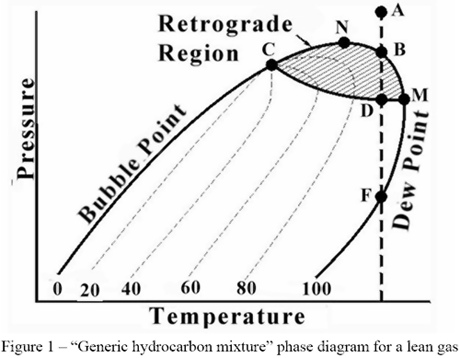Calculating dew point and bubble point of gas mixtures

How do you calculate approximately the triple point and critical point of any given CH-based gas mixture?

I have developed a software which uses iterations to find bubble point and dew point of any CH-based gas mixture. It uses Peng-Robinsson EOS and Van Der Vaals mixing algorithm.

When calculating the dew point pressure for a certain temperature, it's difficult to know the starting range for the iteration. First I used False-Position method in finding the root, but now I have switched to Bisection. Although it's slower, it's more safe as it's not affected by function derivatives.

What would be needed is as approximation of the range where the liquid/vapour phases are found. Setting the iteration's range to start below triple point pressure, or above critical point pressure will cause the iteration to diverge and never find a root.

• Would iterations work with Simplex?
– MaxW
May 17 '19 at 16:59
• What do you use for the liquid phase? May 17 '19 at 21:02
• @MaxW I don't know much about Simplex. Just that it's an optimisation algorithm. I have to look it up. May 19 '19 at 20:31
• @ChetMiller I have calculated the fugacity to a large degree according to this cheguide.com/bubble_p_flash.html May 19 '19 at 20:36
• OK. So you are assuming an ideal liquid solution. May 19 '19 at 22:37In the picture above, the C is the critical point. There seems to be some easy methods to calculate the critical temperature, but not so with the critical pressure.

For bubble point it would give me a good indication of where the max range is.

But I realised that the critical temperature point won't help me finding the min and max range for dew point calculation. As you can see in the picture, the point M (max temperature point) would be a good indication of where the max range is for dew point.

Anyway I found a much simpler solution. I consider the bubble point and dew point calculation functions as a black box.

As I'm only seeking the bubble point / dew point for a specified temperature, I step the possible pressure range (1 kPa - 10 MPa), with steps of 1 kPa. Then I calculate the numerical derivate of using current step and previous step.

This function derivate is consistent and negative in the range of the function domain where a bubble or dew point value is valid.

Outside the function domain the derivate is close to zero (+-1E-15).

So this gives me a good starting point for the root finding algorithm.

I also changed from False Position method to Bisection method, as it's less sensitive to derivates.

I hope this will help someone else with similar problem.